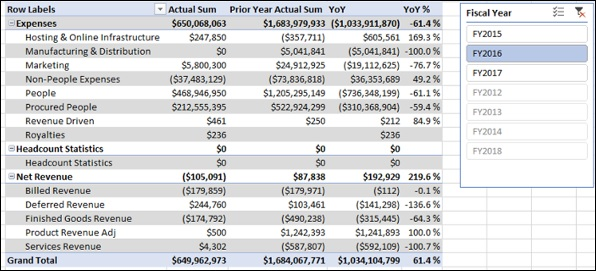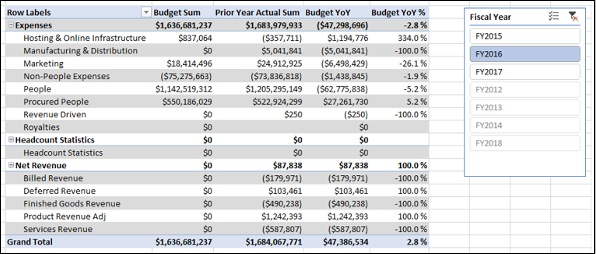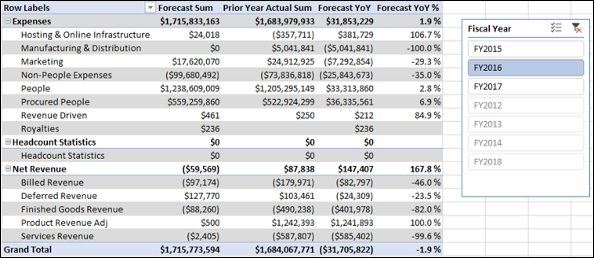# YoY Finance Measures and Analysis# YoY Finance Measures and Analysis

Year-over-Year (YoY) is a measure of growth. It is obtained by subtracting the actual sum of the previous year from the actual sum.

If the result is positive, it reflects an increase in actual, and if it is negative, it reflects a decrease in actual, i.e. if we calculate year-over-year as −

year-over-year = (actual sum –prior year actual sum)

• If the actual sum > the prior year actual sum, year-over-year will be positive.
• If the actual sum < the prior year actual sum, year-over-year will be negative.

In the financial data, accounts such as the expense accounts will have debit (positive) amounts and the revenue accounts will have credit (negative) amounts. Hence, for the expense accounts, the above formula works fine.

However, for the revenue accounts, it should be the reverse, i.e.

• If the actual sum > the prior year actual sum, year-over-year should be negative.
• If the actual sum < the prior year actual sum, year-over-year should be positive.

Hence for the revenue accounts, you have to calculate year-over-year as −

year-over-year = -(actual sum – prior year actual sum)

## Creating Year-over-Year Measure

You can create Year-over-Year measure with the following DAX formula −

YoY:=IF(CONTAINS(Accounts, Accounts[Class],”Net Revenue”),-([Actual Sum]-[Prior Year Actual Sum]), [Actual Sum]-[Prior Year Actual Sum])

In the above DAX formula −

• DAX CONTAINS function returns TRUE, if a row has “Net Revenue” in the column Class in the Accounts table.
• DAX IF function then returns –([Actual Sum]-[ Prior Year Actual Sum]).
• Otherwise, DAX IF function returns [Actual Sum]-[ Prior Year Actual Sum].

## Creating Year-over-Year Percentage Measure

You can represent Year-over-Year as a percentage with the ratio −

(YoY) / (Prior Year Actual Sum)

You can create the Year-over-Year Percentage measure with the following DAX formula −

YoY %:=IF([Prior Year Actual Sum], [YoY] / ABS([Prior Year Actual Sum]),BLANK())

DAX IF function is used in the above formula to ensure that there is no division by zero.

## Analyzing Data with Year-over-Year Measures

Create a Power PivotTable as follows −

• Add the fields Class and Sub Class from the Accounts table to Rows.
• Add the measures – Actual Sum, Prior Year Actual Sum, YoY and YoY % to Values.
• Insert a Slicer on the field Fiscal Year from the Date table.
• Select FY2016 in the Slicer.## Creating Budget Year-over-Year Measure

You can create Budget Year-over-Year measure as follows −

Budget YoY: = IF(CONTAINS(Accounts,Accounts[Class],”Net Revenue”), – ([Budget Sum] – [Prior Year Actual Sum]), [Budget Sum] – [Prior Year Actual Sum])

## Creating Budget Year-over-Year Percentage Measure

You can create Budget Year-over-Year Percentage measure as follows −

Budget YoY %:=IF([Prior Year Actual Sum] , [Budget YoY] / ABS ([Prior Year Actual Sum]) , BLANK())

## Analyzing Data with Budget Year-over-Year Measures

Create a Power PivotTable as follows −

• Add the fields Class and Sub Class from the Accounts table to Rows.
• Add the measures – Budget Sum, Prior Year Actual Sum, Budget YoY and Budget YoY % to Values.
• Insert a Slicer on the field Fiscal Year from the Date table.
• Select FY2016 in the Slicer.## Creating Forecast Year-over-Year Measure

You can create Forecast Year-over-Year measure as follows −

Forecast YoY:=IF(CONTAINS(Accounts,Accounts[Class],”Net Revenue”), – ([Forecast Sum] – [Prior Year Actual Sum]), [Forecast Sum] – [Prior Year Actual Sum])

## Creating Forecast Year-over-Year Percentage Measure

You can create Forecast Year-over-Year Percentage measure as follows −

Forecast YoY %:=IF([Prior Year Actual Sum],[Forecast YoY]/ABS([Prior Year Actual Sum]),BLANK())

## Analyzing Data with Forecast Year-over-Year Measures

Create a Power PivotTable as follows −

• Add the fields Class and Sub Class from the Accounts table to Rows.
• Add the measures – Forecast Sum, Prior Year Actual Sum, Forecast YoY and Forecast YoY % to Values.
• Insert a Slicer on the field Fiscal Year from the Data table.
• Select FY2016 in the Slicer.